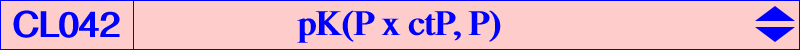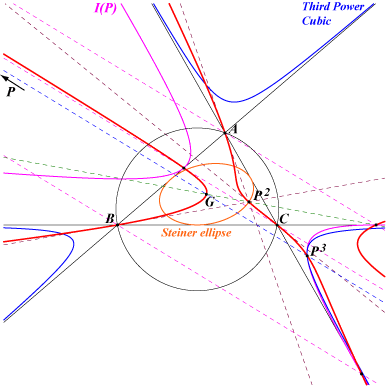Let P be a point and I(P) the inconic with perspector P and center ctP, the complement of the isotomic conjugate of P. We consider the pivotal isocubic K(P) with pivot P which is invariant in the isoconjugation which swaps P and P* = ctP. K(P) is also the locus of point M such that P*, M and P/M are collinear (P* is the isopivot or secondary pivot). Hence, any line L passing through P* meets the cubic at two points such that the bicevian conic C(M, P/M) is bitangent to I(P) at two points Z1, Z2 on the line L which are obviously antipodes on I(P). With P = u : v : w, the equation of K(P) is : u^2(v+w)(vz-wy)yz + cyclic = 0. Points on the curve : the pivot P and the vertices Pa, Pb, Pc of the cevian triangle of P. the isopivot P* = ctP. the pole W = P x P* which is the cevian quotient P/P*; the centroid G which is W*. the cevian quotient P/G which is atP, the anticomplement of the isotomic conjugate of P. Remarks : The tangents at P*, Pa, Pb, Pc pass through W, the pole of the isoconjugation. The tangents at P, A, B, C pass through P*, the center of I(P). The following table gives a selection of such cubics.P W cubic or X(i) on the cubic for i = remarks X(1) X(42) 1, 2, 37, 42, 192 X(3) X(418) 2, 3, 216, 418 X(4) X(25) K233 I(P) is the ellipse K X(6) X(3051) K368 I(P) is the Brocard ellipse X(7) X(57) K365 I(P) is the incircle X(8) X(200) 2, 8, 9, 144, 200 I(P) is the Mandart ellipse X(69) X(394) K099 X(75) X(321) K366 X(99) X(2) K242 I(P) is the Kiepert parabola X(264) X(324) K674 I(P) is the McBeath inconic X(648) X(2) 2, 525, 648, 2479, 2480, 2592, 2593 X(886) X(2) 2, 512, 538, 670, 886, 888 X(889) X(2) 2, 513, 536, 668, 889, 891 X(892) X(2) K240Special cases P on the Steiner ellipse A cubic K(P) with P on the Steiner ellipse is an isotomic cubic and I(P) is a parabola. K(P) meets the line at infinity at P* and two other points on the circum-conic with perspector P*. K(P) meets the Steiner ellipse at P and two other points on the trilinear polar of P which are antipodes on the ellipse. See K242 and K240 for examples.   P at infinity An cubic K(P) with P on the line at infinity has its isopivot P^2 on the Steiner in-ellipse and its pole is the barycentric cube P^3 of P. These cubics are quite remarkable since they contain five powers of P namely P^0 = G, P^1 = P, P^2 (isopivot), P^3 (pole), P^(3/2) (real or not fixed point of the isoconjugation) and its harmonic associates.The barycentric square M^2 of a point M is the pole of G in the pencil of conics passing through M and the vertices of the anticevian triangle of M and the barycentric cube M^3 of M is the pole of its isotomic conjugate in the same pencil. The Steiner in-ellipse is the set of barycentric squares of the points at infinity. The third power cubic is the set of barycentric cubes of the points at infinity. It is the homothetic of the Tucker nodal cubic K015 under h(G,3/2). As such, its is also an unicursal cubic with singularity at G.K(P)+ The cubic is a K+ if and only if P lies on the nK(X2, X2) with equation : 3[x(y-z)^2 + cyclic] + 26 xyz = 0.